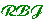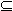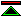# Arithmetisation of metamathematics in a general setting

###'s Introduction and Conundrum

 These notes are going to be exceptionally bizarre even by my standards, because I have read only a tiny part of this paper and its mostly pretty much beyond my ken. I'm going to explain why it may be relevant to one of my purposes and maybe there is someone out there who will find this faintly interesting. I can't remember how or why I came into possession of a photocopy of this paper, but sometime after this happened it dawned on me that it might have something in it which is relevant to a problem that I have meditated upon, on and off, for decades (since at least 1985). The problem is, how to get logical foundation systems for mathematics which are radically different to classical set theory (in particular, which are "non-well-founded", and not formulated as first order theories, though that isn't the whole story) but which are just as strong as (preferably a touch stronger than), say, ZFC. In about 1987 I began to half understand "strong" as in "proof theoretic strength", which I took to be an ordering induced by relative consistency proofs and shown to be non-trivial by Gödel's second incompleteness theorem. Now at some point I decided that one interesting way of getting strength is simply to steal it. You start off with a language with the right kind of semantics (which is itself a tricky story but won't detain me here), and then you start the proof system in a very cautious way, so that you get a weak foundation system in which you can have reasonable confidence of soundness and consistency. Lets suppose that you have done this and you get something equivalent in strength to Peano Arithmetic. You then steal the rest of the strength needed to do something like full classical mathematics by adding an axiom asserting the consistency of ZFC. Now, for present purposes let us put aside the question of whether this will yield a pragmatically convenient foundation system (which seems highly improbable), and just focus on the question of whether this works at all, by which I mean, does it yeild a consistent logical foundation with proof theoretic strength greater than ZFC. Clearly it will work in some cases (e.g. you start with ZFC and then add the claim that ZFC is consistent, and you end up with something stronger than ZFC), and it will fail in some other cases (e.g. you start with the language of first order arithmetic but no non-logical axioms and then add the axiom which asserts the consistency of ZFC as the sole non-logical axiom, giving something which I would guess is not much use even in principle, let alone in practice). So really the question is, under what conditions will it work?

### Feferman provides the answer?

This paper by Feferman seems to provide the answer, that it works if the theory you start with is as strong as PA. Now I can't be sure about that, because I'm just not that good a logician. I havn't actually read the paper properly, and if I had I still wouldn't have fully understood it.

It looks like its a corollary of:

 Theorem 6.2 let A= and S be axiom systems, where PS. Suppose that a numerates A in S. Then A is interpretable in S + {Con(a)}.

Where P is the peano axioms.

There is the problem that this is just about first order theories and so I have to guess whether there is any analogous result for logics in general.

###'s second conjecture

(the first one was that its possible to steal proof theoretic strength)
 This is how I really got to be writing these notes. I was reading this paper by Hartry Field ([Field97]) in which he dismisses a number of Gödelian arguments against extreme anti-objectivism concerning arithmetic. It seemed to me that Gödelian arguments, even if they work, are overkill. For me, first port of call (in defence of the objectivity of truth in arithmetic) is just arguing that the natural numbers and the successor operation form a well understood and uniquely defined structure. However, if you want something more, my next port of call would be w-consistency. Clearly an w-inconsistent extension of PA is not worth considering (i.e. its not really about the natural numbers any more, you've thrown away the intended interpretation). So the question arises, how much does this buy you? And I conjectured "everything" (i.e. true arithmetic is the only w-consistent completion of Peano arithmetic). And then I decided that this same result of Feferman (Theorem 6.2) could be used to prove it. I think I was wrong, but that made me include the reference to the paper, and then these notes. The conjecture and the incorrect "proof" of it using Theorem 6.2. is here.©created 1998/10/16 modified 1998/10/16